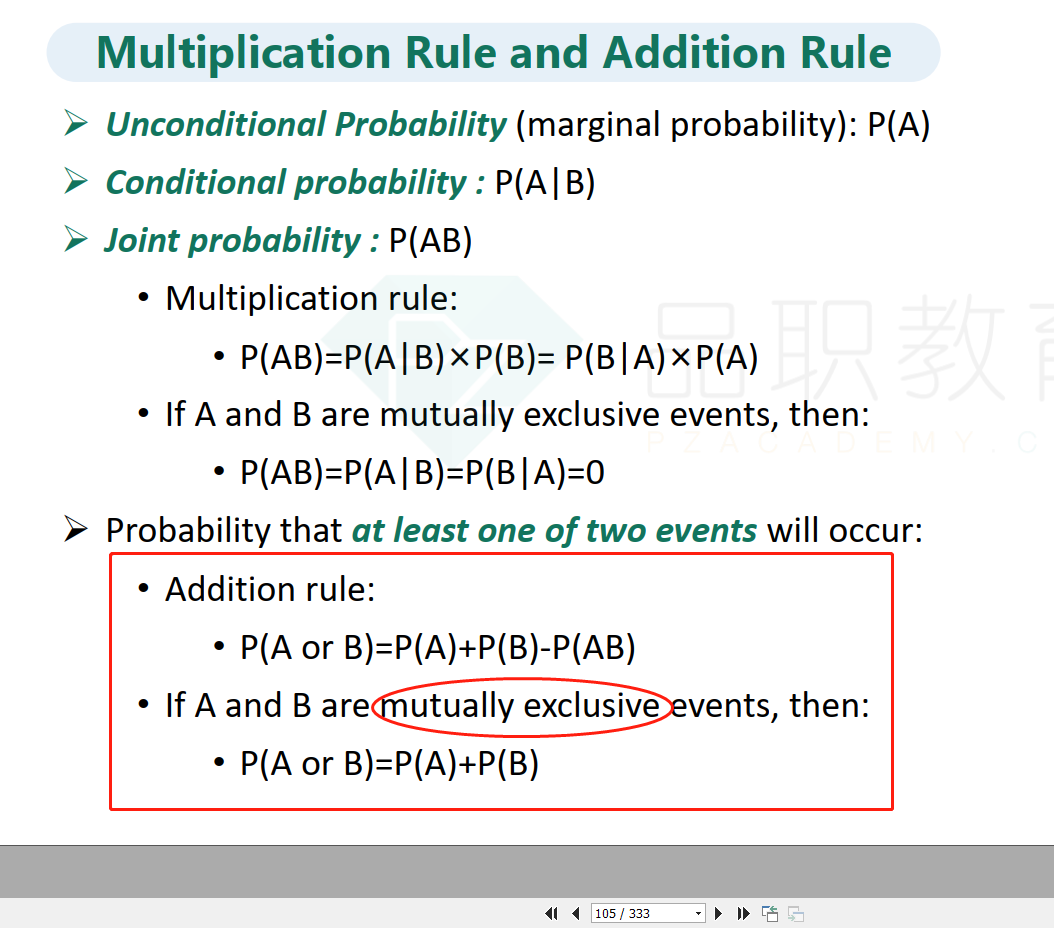Yuri · 2022年11月29日

# 问

NO.PZ2015120604000067

Which of the following statements is the most correct?

A.

If events A and B are mutually exclusive, then $\mathrm{P\left(}$A or B)=P(A)-P(B)

B.

If events A and B are mutually exclusive, then $\mathrm{P\left(}\left(A\mathrm{B\right)}\right)=\mathrm{P\left(}\left(\mathrm{A\right)}\right)×\mathrm{P\left(}\left(\mathrm{B\right)}\right)$.

C.

If events A and B are mutually exclusive, then $\mathrm{P\left(}\left(Aor\mathrm{B\right)}\right)=\mathrm{P\left(}\left(\mathrm{A\right)}\right)+\mathrm{P\left(}\left(\mathrm{B\right)}\right)$

C is correct.

$\mathrm{P\left(}\left(A\mathrm{B\right)}\right)=\mathrm{P\left(}\left(\mathrm{A\right)}\right)×\mathrm{P\left(}\left(\mathrm{B\right)}\right)$ is only correct when A and B are independent events. In this case, $\mathrm{P\left(}\left(A/\mathrm{B\right)}\right)=0$,$\mathrm{P\left(}\left(A\mathrm{B\right)}\right)=0$，because event A and B are mutually exclusive

###### 1 个答案1）根据加法法则，P(A or B)=P(A)+P(B)-P(AB)；

2）由于“events A and B are mutually exclusive”，所以P(AB)=0；